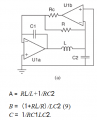# help me with nodal analysis

#### farah123

Joined May 11, 2018
3
Dear all

I’m trying to understand chau circuit in chaotic system, one of the proposed CLC circuit with parameters attached bellow showed a final parameters of the circuit. Can anyone help me with the nodal analysis of these parameters? How the researcher derived them?A = RL/L+1/RC2

B = (1+RL/R)/LC2 (9)

C = 1/RC1LC2.

#### Attachments

• 169.6 KB Views: 7

#### WBahn

Joined Mar 31, 2012
28,488
You image file didn't post. Assuming it's the contents of the Word file, here it is:I don't know what A, B, and C are supposed to represent, but the first two are fundamentally meaningless because they are dimensionally inconsistent.

The first term on the right in the A equation has units of resistance while the second term has units of inverse time (assuming the C2 is supposed to be in the denominator).

The numerator of the B term tries to add inductance to a dimensionless quantity.

So these two equations, at the very least, are wrong.

What's the (9) supposed to mean?

What is this circuit supposed to do? You mention a chaotic system. Is this circuit supposed to produce chaotic results? If so, then it must be operating non-linearly. If that's the case, then far more information is probably needed about those opamps.

When all you circuit does is provide an opamp symbol, there is little choice but to assume that it is operating linearly, especially since the rail voltages aren't even given.

Assuming they are linear, try identifying which nodes in that circuit that are clamped at specific voltages.

#### farah123

Joined May 11, 2018
3
You image file didn't post. Assuming it's the contents of the Word file, here it is:

View attachment 152394

I don't know what A, B, and C are supposed to represent, but the first two are fundamentally meaningless because they are dimensionally inconsistent.

The first term on the right in the A equation has units of resistance while the second term has units of inverse time (assuming the C2 is supposed to be in the denominator).

The numerator of the B term tries to add inductance to a dimensionless quantity.

So these two equations, at the very least, are wrong.

What's the (9) supposed to mean?

What is this circuit supposed to do? You mention a chaotic system. Is this circuit supposed to produce chaotic results? If so, then it must be operating non-linearly. If that's the case, then far more information is probably needed about those opamps.

When all you circuit does is provide an opamp symbol, there is little choice but to assume that it is operating linearly, especially since the rail voltages aren't even given.

Assuming they are linear, try identifying which nodes in that circuit that are clamped at specific voltages.

Thanks for your reply. here is the original paper and the mentioned circuit is on page 733.I will be very thankful if you could help me.
https://www.researchgate.net/publication/224170502_Simple_Autonomous_Chaotic_Circuits

#### WBahn

Joined Mar 31, 2012
28,488
Thanks for your reply. here is the original paper and the mentioned circuit is on page 733.I will be very thankful if you could help me.
https://www.researchgate.net/publication/224170502_Simple_Autonomous_Chaotic_Circuits
Have you walked through, line by line, of the derivation provided in that article?

If not, then that should be your next step.

Come back and focus on the first point where you can't figure out how they went from one step to the next. That will be far more effective than asking how they arrived at essentially the final conclusion.

#### farah123

Joined May 11, 2018
3